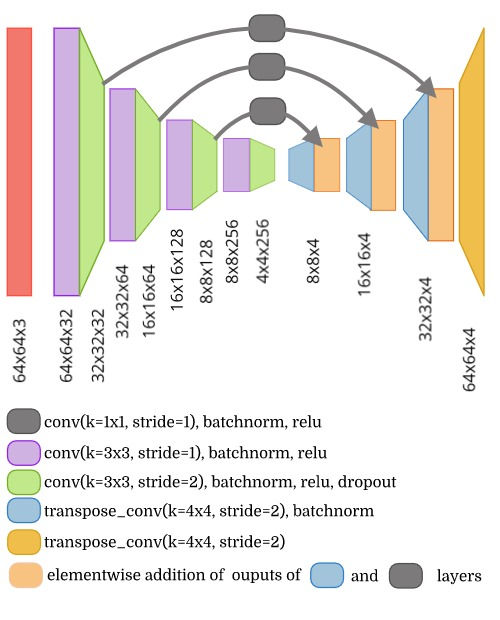# Get Hands on - Model Overview

We will use a simple model that uses the architecture shown in the diagram below. For the downsampling half of the architecture, it goes through pairs of convolutions. The first with a stride of 1, and the second with a stride of 2 to downsample by half. In addition to the standard connections you would usually see in a convolutional neural network, there are also some skip connections that branch out from the second convolution of each pair. This connection goes through a 1x1 convolution, which is added to the outputs of layers further ahead.In the upsampling half, transpose convolutions with a kernel size of 4, and stride of 2 are used to upsample. The output of this goes through an elementwise addition with the output of the skip connections. Each of the transpose convolutions has batch normalization applied to them, except for the very final one, which provides us with the output logits.